# RD Sharma Solutions for Class 10 Maths Chapter 2 Polynomials Exercise 2.1

The basic study of polynomials and their geometrical representation of linear and quadratic polynomials along with the geometrical meaning of their zeros are the main insights of this chapter. The RD Sharma Solutions Class 10 Exercise 2.1, contains problems dealing with finding the zeros of polynomials and verification of the relationship between the zeros and their coefficients. In order for students to excel in the exercise questions and boost their confidence, the RD Sharma Solutions for Class 10 Maths Chapter 2 Polynomials Exercise 2.1 PDF is provided below.

## RD Sharma Solutions for Class 10 Maths Chapter 2 Polynomials Exercise 2.1 Download PDF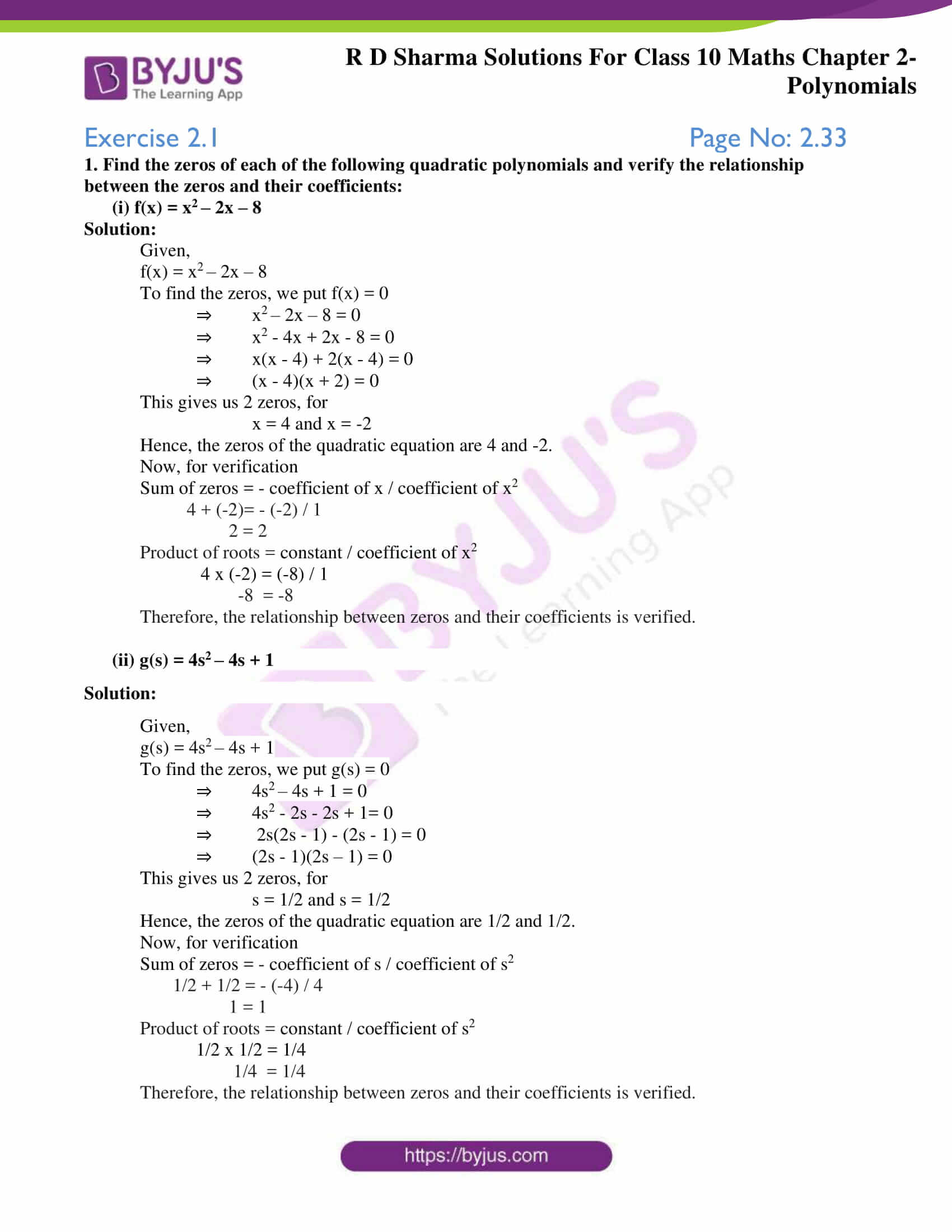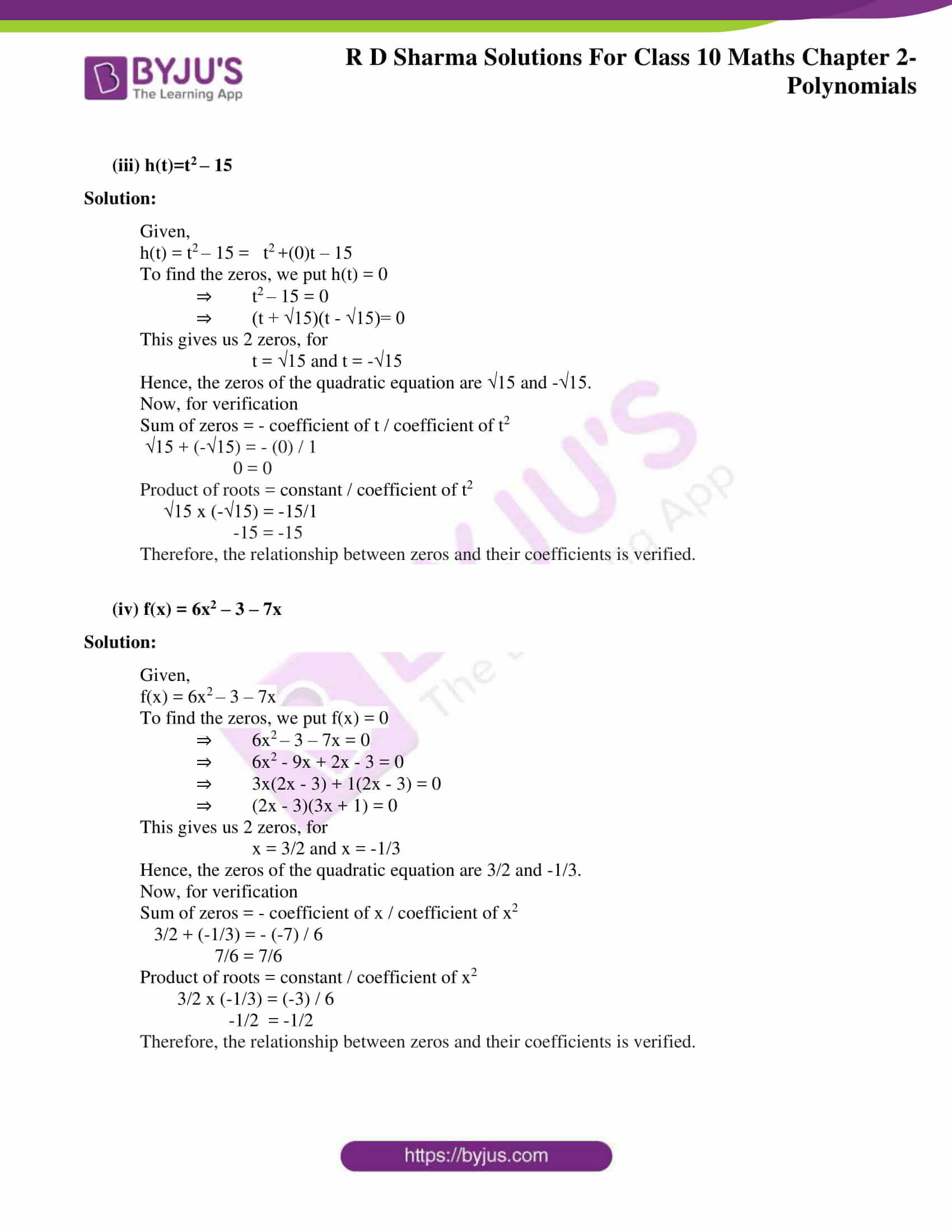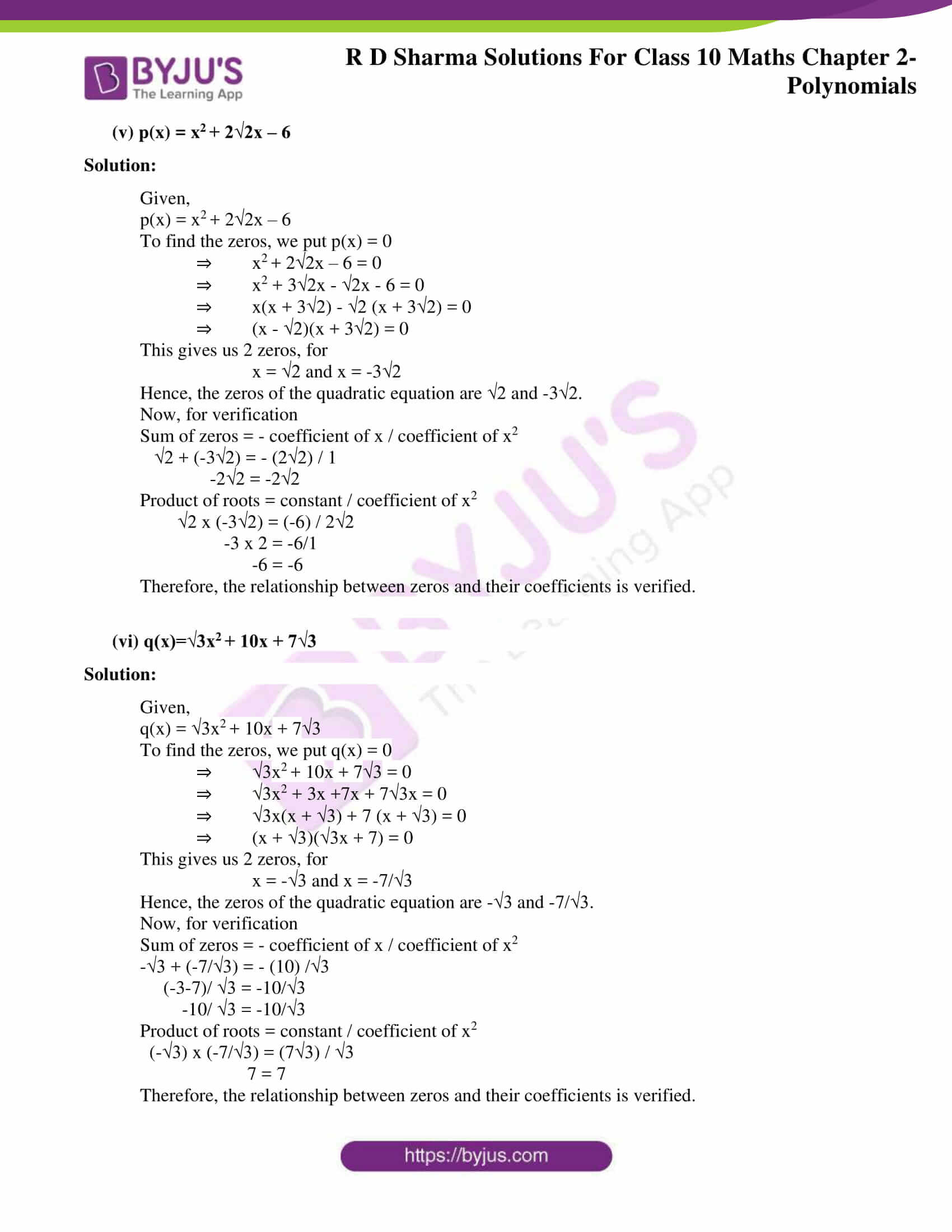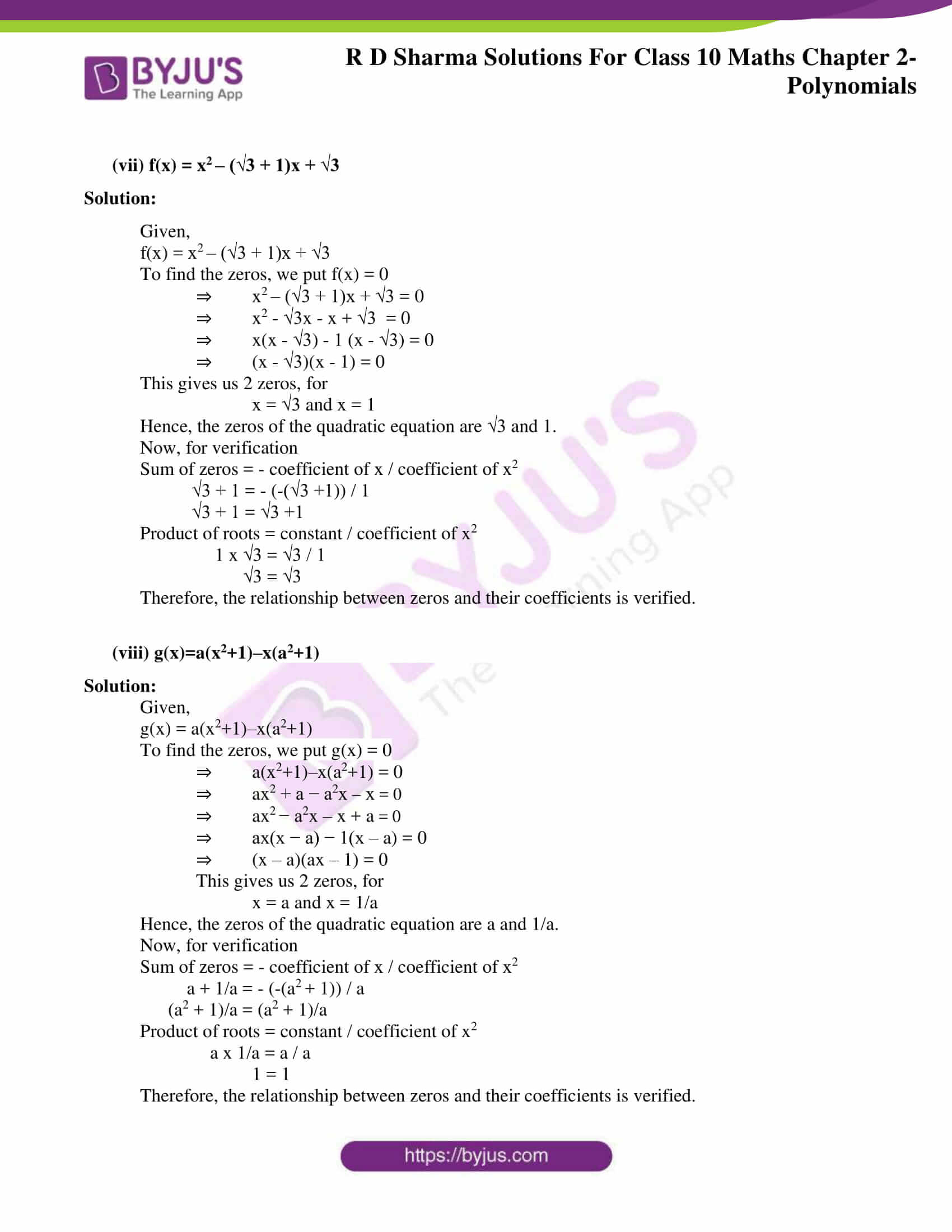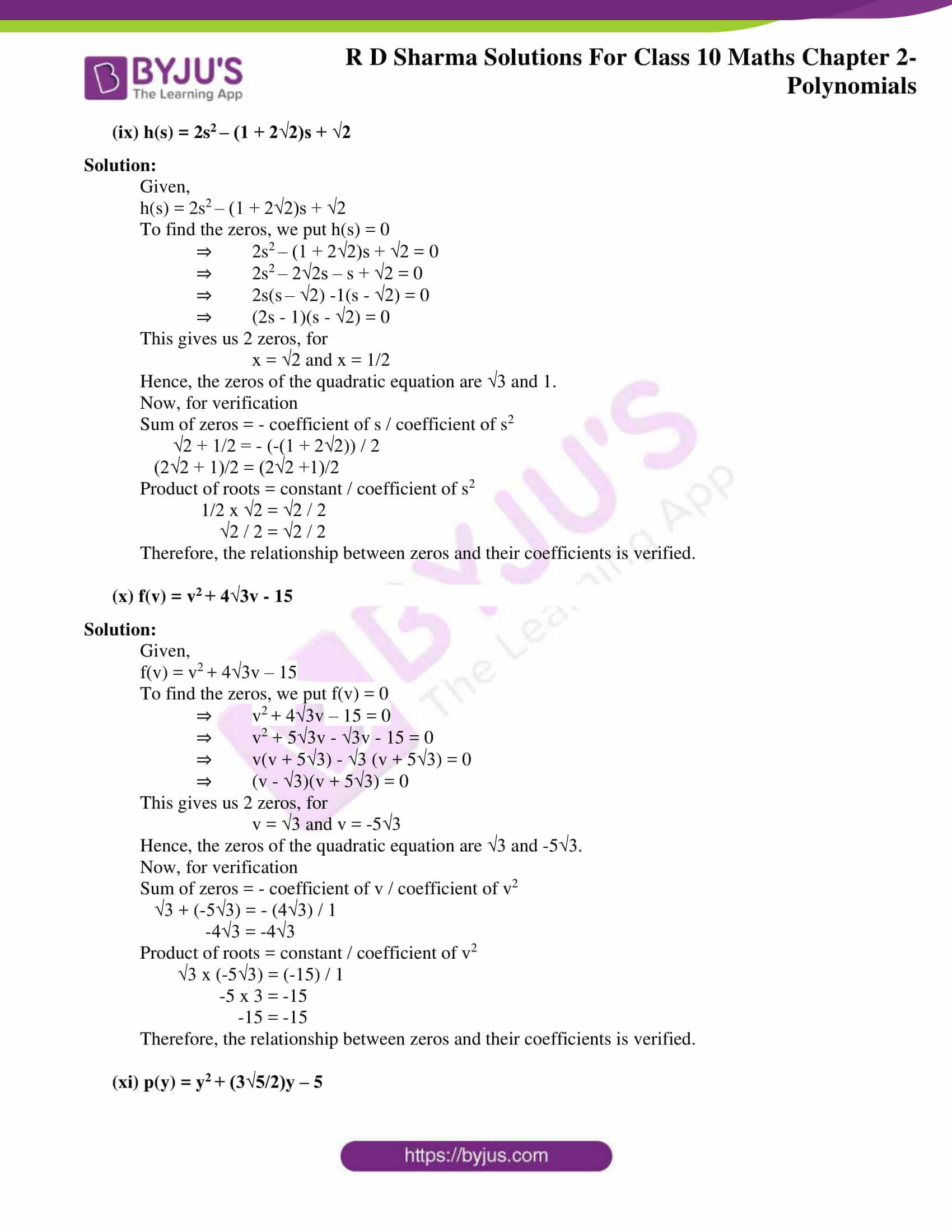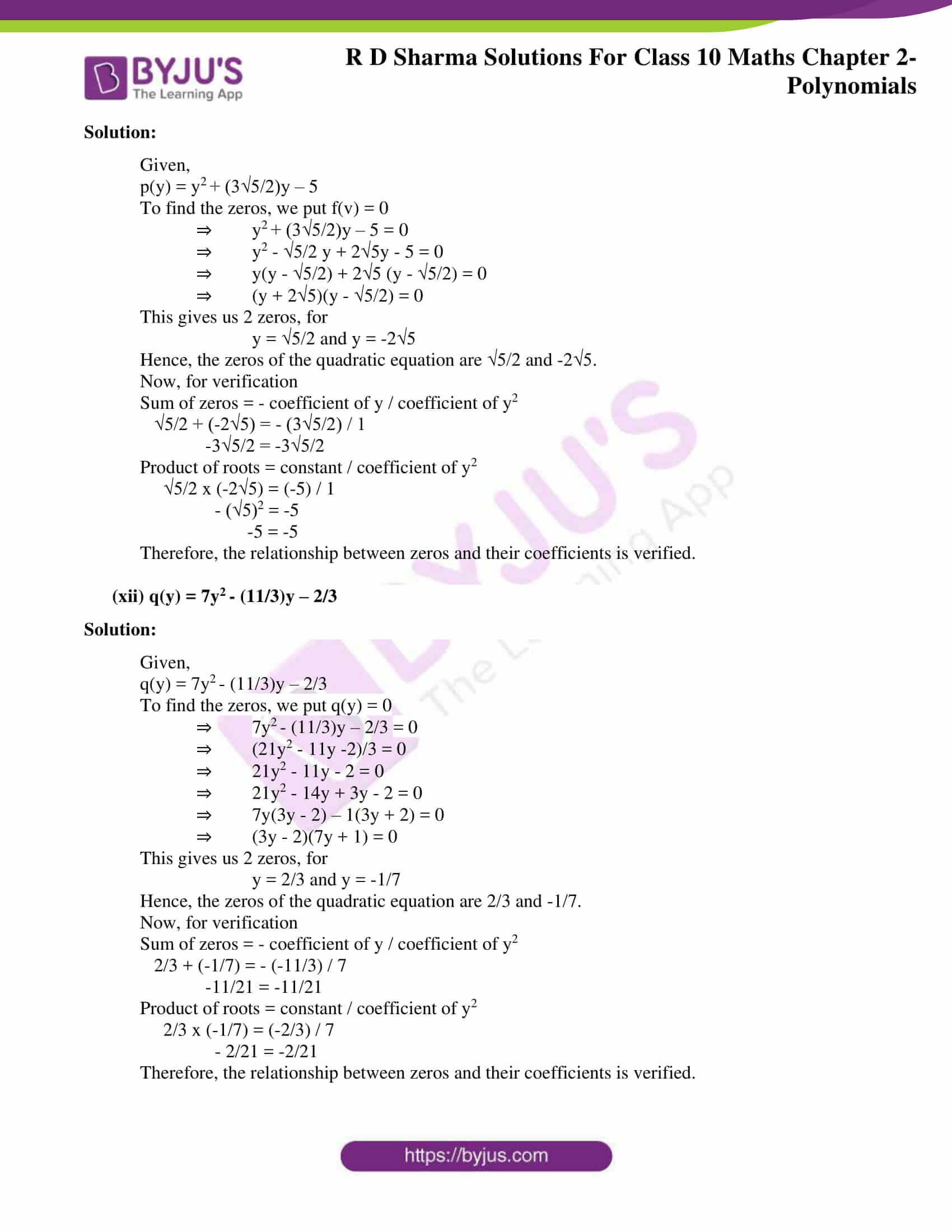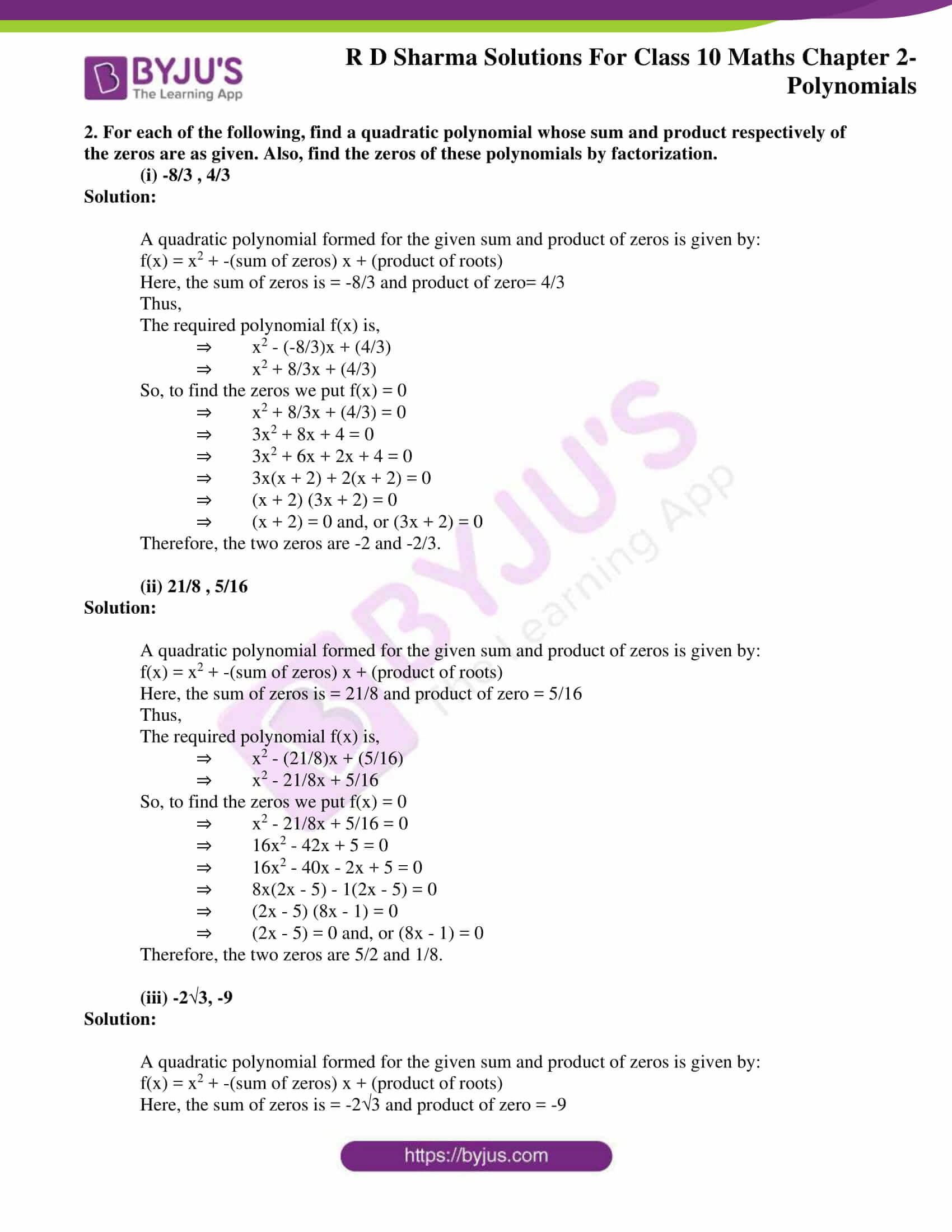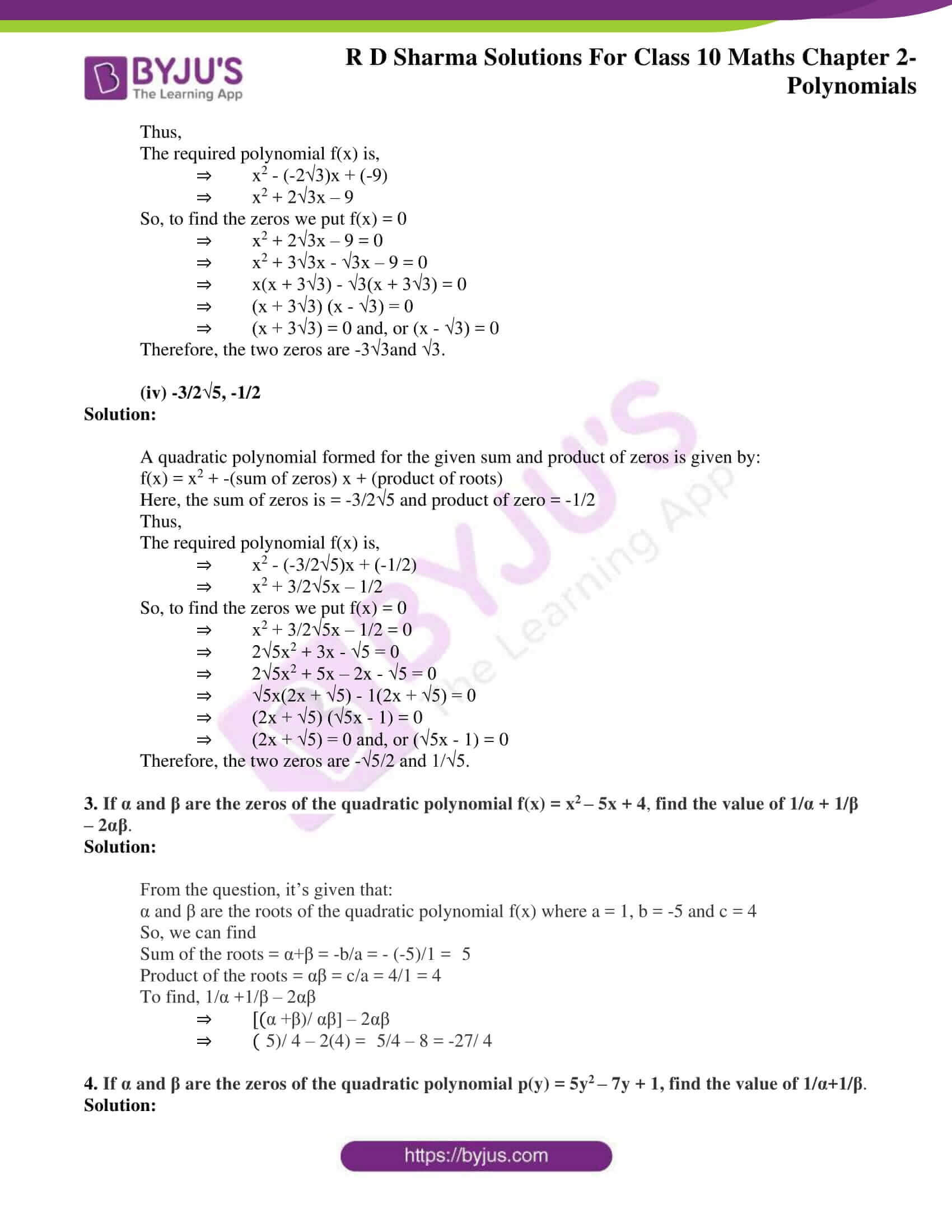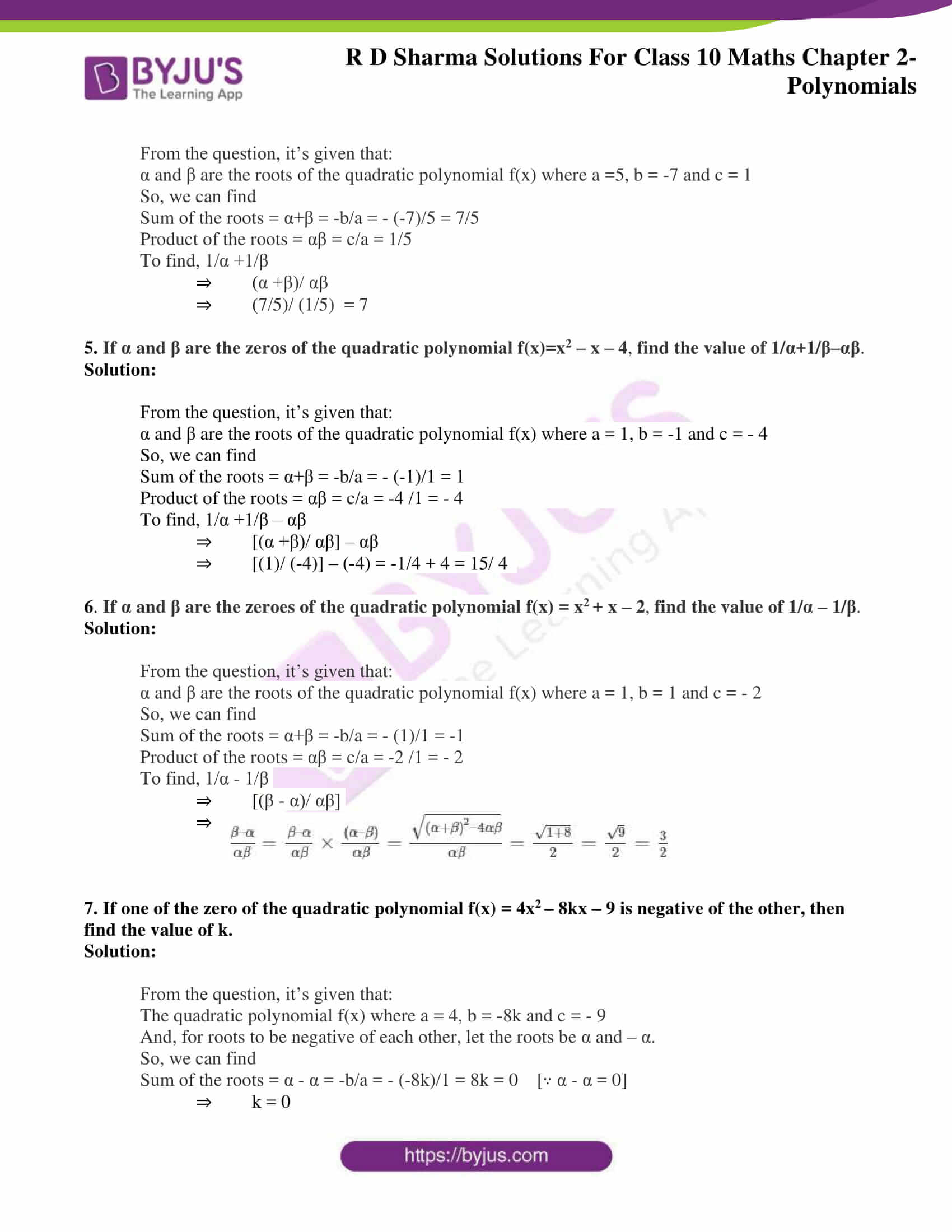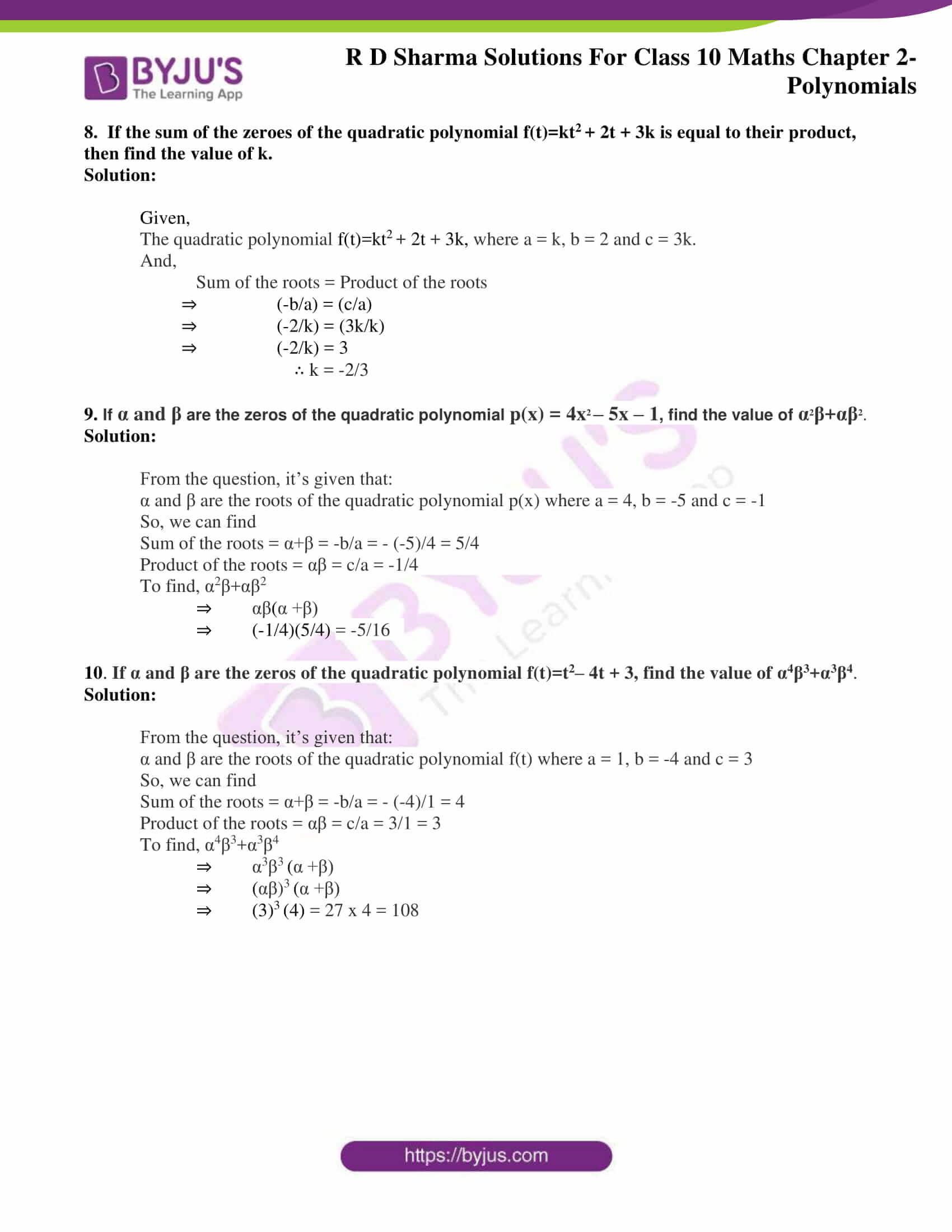### Access RD Sharma Solutions for Class 10 Maths Chapter 2 Polynomials Exercise 2.1

1. Find the zeros of each of the following quadratic polynomials and verify the relationship between the zeros and their coefficients:

(i) f(x) = x– 2x – 8

Solution:

Given,

f(x) = x– 2x – 8

To find the zeros, we put f(x) = 0

⇒ x– 2x – 8 = 0

⇒  x2 – 4x + 2x – 8 = 0

⇒ x(x – 4) + 2(x – 4) = 0

⇒ (x – 4)(x + 2) = 0

This gives us 2 zeros, for

x = 4 and x = -2

Hence, the zeros of the quadratic equation are 4 and -2.

Now, for verification

Sum of zeros = – coefficient of x / coefficient of x2

4 + (-2)= – (-2) / 1

2 = 2

Product of roots = constant / coefficient of x2

4 x (-2) = (-8) / 1

-8 = -8

Therefore, the relationship between zeros and their coefficients is verified.

(ii) g(s) = 4s– 4s + 1

Solution:

Given,

g(s) = 4s– 4s + 1

To find the zeros, we put g(s) = 0

⇒ 4s– 4s + 1 = 0

⇒  4s2 – 2s – 2s + 1= 0

⇒  2s(2s – 1) – (2s – 1) = 0

⇒ (2s – 1)(2s – 1) = 0

This gives us 2 zeros, for

s = 1/2 and s = 1/2

Hence, the zeros of the quadratic equation are 1/2 and 1/2.

Now, for verification

Sum of zeros = – coefficient of s / coefficient of s2

1/2 + 1/2 = – (-4) / 4

1 = 1

Product of roots = constant / coefficient of s2

1/2 x 1/2 = 1/4

1/4 = 1/4

Therefore, the relationship between zeros and their coefficients is verified.

(iii) h(t)=t– 15

Solution:

Given,

h(t) = t– 15 = t+(0)t – 15

To find the zeros, we put h(t) = 0

⇒ t– 15 = 0

⇒ (t + √15)(t – √15)= 0

This gives us 2 zeros, for

t = √15 and t = -√15

Hence, the zeros of the quadratic equation are √15 and -√15.

Now, for verification

Sum of zeros = – coefficient of t / coefficient of t2

√15 + (-√15) = – (0) / 1

0 = 0

Product of roots = constant / coefficient of t2

√15 x (-√15) = -15/1

-15 = -15

Therefore, the relationship between zeros and their coefficients is verified.

(iv) f(x) = 6x2 – 3 – 7x

Solution:

Given,

f(x) = 6x– 3 – 7x

To find the zeros, we put f(x) = 0

⇒ 6x– 3 – 7x = 0

⇒  6x2 – 9x + 2x – 3 = 0

⇒ 3x(2x – 3) + 1(2x – 3) = 0

⇒ (2x – 3)(3x + 1) = 0

This gives us 2 zeros, for

x = 3/2 and x = -1/3

Hence, the zeros of the quadratic equation are 3/2 and -1/3.

Now, for verification

Sum of zeros = – coefficient of x / coefficient of x2

3/2 + (-1/3) = – (-7) / 6

7/6 = 7/6

Product of roots = constant / coefficient of x2

3/2 x (-1/3) = (-3) / 6

-1/2 = -1/2

Therefore, the relationship between zeros and their coefficients is verified.

(v) p(x) = x+ 2√2x – 6

Solution:

Given,

p(x) = x+ 2√2x – 6

To find the zeros, we put p(x) = 0

⇒ x+ 2√2x – 6 = 0

⇒  x2 + 3√2x – √2x – 6 = 0

⇒ x(x + 3√2) – √2 (x + 3√2) = 0

⇒ (x – √2)(x + 3√2) = 0

This gives us 2 zeros, for

x = √2 and x = -3√2

Hence, the zeros of the quadratic equation are √2 and -3√2.

Now, for verification

Sum of zeros = – coefficient of x / coefficient of x2

√2 + (-3√2) = – (2√2) / 1

-2√2 = -2√2

Product of roots = constant / coefficient of x2

√2 x (-3√2) = (-6) / 2√2

-3 x 2 = -6/1

-6 = -6

Therefore, the relationship between zeros and their coefficients is verified.

(vi) q(x)=√3x+ 10x + 7√3

Solution:

Given,

q(x) = √3x+ 10x + 7√3

To find the zeros, we put q(x) = 0

⇒ √3x+ 10x + 7√3 = 0

⇒  √3x2 + 3x +7x + 7√3x = 0

⇒ √3x(x + √3) + 7 (x + √3) = 0

⇒ (x + √3)(√3x + 7) = 0

This gives us 2 zeros, for

x = -√3 and x = -7/√3

Hence, the zeros of the quadratic equation are -√3 and -7/√3.

Now, for verification

Sum of zeros = – coefficient of x / coefficient of x2

-√3 + (-7/√3) = – (10) /√3

(-3-7)/ √3 = -10/√3

-10/ √3 = -10/√3

Product of roots = constant / coefficient of x2

(-√3) x (-7/√3) = (7√3) / √3

7 = 7

Therefore, the relationship between zeros and their coefficients is verified.

(vii) f(x) = x– (√3 + 1)x + √3

Solution:

Given,

f(x) = x– (√3 + 1)x + √3

To find the zeros, we put f(x) = 0

⇒ x– (√3 + 1)x + √3 = 0

⇒  x2 – √3x – x + √3 = 0

⇒ x(x – √3) – 1 (x – √3) = 0

⇒ (x – √3)(x – 1) = 0

This gives us 2 zeros, for

x = √3 and x = 1

Hence, the zeros of the quadratic equation are √3 and 1.

Now, for verification

Sum of zeros = – coefficient of x / coefficient of x2

√3 + 1 = – (-(√3 +1)) / 1

√3 + 1 = √3 +1

Product of roots = constant / coefficient of x2

1 x √3 = √3 / 1

√3 = √3

Therefore, the relationship between zeros and their coefficients is verified.

(viii) g(x)=a(x2+1)–x(a2+1)

Solution:

Given,

g(x) = a(x2+1)–x(a2+1)

To find the zeros, we put g(x) = 0

⇒ a(x2+1)–x(a2+1) = 0

⇒ ax2 + a − a2x – x = 0

⇒ ax− a2x – x + a = 0

⇒ ax(x − a) − 1(x – a) = 0

⇒ (x – a)(ax – 1) = 0

This gives us 2 zeros, for

x = a and x = 1/a

Hence, the zeros of the quadratic equation are a and 1/a.

Now, for verification

Sum of zeros = – coefficient of x / coefficient of x2

a + 1/a = – (-(a2 + 1)) / a

(a2 + 1)/a = (a2 + 1)/a

Product of roots = constant / coefficient of x2

a x 1/a = a / a

1 = 1

Therefore, the relationship between zeros and their coefficients is verified.

(ix) h(s) = 2s– (1 + 2√2)s + √2

Solution:

Given,

h(s) = 2s– (1 + 2√2)s + √2

To find the zeros, we put h(s) = 0

⇒ 2s– (1 + 2√2)s + √2 = 0

⇒  2s– 2√2s – s + √2 = 0

⇒ 2s(s – √2) -1(s – √2) = 0

⇒ (2s – 1)(s – √2) = 0

This gives us 2 zeros, for

x = √2 and x = 1/2

Hence, the zeros of the quadratic equation are √3 and 1.

Now, for verification

Sum of zeros = – coefficient of s / coefficient of s2

√2 + 1/2 = – (-(1 + 2√2)) / 2

(2√2 + 1)/2 = (2√2 +1)/2

Product of roots = constant / coefficient of s2

1/2 x √2 = √2 / 2

√2 / 2 = √2 / 2

Therefore, the relationship between zeros and their coefficients is verified.

(x) f(v) = v+ 4√3v – 15

Solution:

Given,

f(v) = v+ 4√3v – 15

To find the zeros, we put f(v) = 0

⇒ v+ 4√3v – 15 = 0

⇒  v2 + 5√3v – √3v – 15 = 0

⇒ v(v + 5√3) – √3 (v + 5√3) = 0

⇒ (v – √3)(v + 5√3) = 0

This gives us 2 zeros, for

v = √3 and v = -5√3

Hence, the zeros of the quadratic equation are √3 and -5√3.

Now, for verification

Sum of zeros = – coefficient of v / coefficient of v2

√3 + (-5√3) = – (4√3) / 1

-4√3 = -4√3

Product of roots = constant / coefficient of v2

√3 x (-5√3) = (-15) / 1

-5 x 3 = -15

-15 = -15

Therefore, the relationship between zeros and their coefficients is verified.

(xi) p(y) = y+ (3√5/2)y – 5

Solution:

Given,

p(y) = y+ (3√5/2)y – 5

To find the zeros, we put f(v) = 0

⇒ y+ (3√5/2)y – 5 = 0

⇒  y2 – √5/2 y + 2√5y – 5 = 0

⇒ y(y – √5/2) + 2√5 (y – √5/2) = 0

⇒ (y + 2√5)(y – √5/2) = 0

This gives us 2 zeros, for

y = √5/2 and y = -2√5

Hence, the zeros of the quadratic equation are √5/2 and -2√5.

Now, for verification

Sum of zeros = – coefficient of y / coefficient of y2

√5/2 + (-2√5) = – (3√5/2) / 1

-3√5/2 = -3√5/2

Product of roots = constant / coefficient of y2

√5/2 x (-2√5) = (-5) / 1

– (√5)2 = -5

-5 = -5

Therefore, the relationship between zeros and their coefficients is verified.

(xii) q(y) = 7y– (11/3)y – 2/3

Solution:

Given,

q(y) = 7y– (11/3)y – 2/3

To find the zeros, we put q(y) = 0

⇒ 7y– (11/3)y – 2/3 = 0

⇒  (21y2 – 11y -2)/3 = 0

⇒ 21y2 – 11y – 2 = 0

⇒ 21y2 – 14y + 3y – 2 = 0

⇒ 7y(3y – 2) – 1(3y + 2) = 0

⇒ (3y – 2)(7y + 1) = 0

This gives us 2 zeros, for

y = 2/3 and y = -1/7

Hence, the zeros of the quadratic equation are 2/3 and -1/7.

Now, for verification

Sum of zeros = – coefficient of y / coefficient of y2

2/3 + (-1/7) = – (-11/3) / 7

-11/21 = -11/21

Product of roots = constant / coefficient of y2

2/3 x (-1/7) = (-2/3) / 7

– 2/21 = -2/21

Therefore, the relationship between zeros and their coefficients is verified.

2. For each of the following, find a quadratic polynomial whose sum and product respectively of the zeros are as given. Also, find the zeros of these polynomials by factorization.

(i) -8/3 , 4/3

Solution:

A quadratic polynomial formed for the given sum and product of zeros is given by:

f(x) = x2 + -(sum of zeros) x + (product of roots)

Here, the sum of zeros is = -8/3 and product of zero= 4/3

Thus,

The required polynomial f(x) is,

⇒ x2 – (-8/3)x + (4/3)

⇒ x2 + 8/3x + (4/3)

So, to find the zeros we put f(x) = 0

⇒ x2 + 8/3x + (4/3) = 0

⇒ 3x2 + 8x + 4 = 0

⇒ 3x2 + 6x + 2x + 4 = 0

⇒ 3x(x + 2) + 2(x + 2) = 0

⇒ (x + 2) (3x + 2) = 0

⇒ (x + 2) = 0 and, or (3x + 2) = 0

Therefore, the two zeros are -2 and -2/3.

(ii) 21/8 , 5/16

Solution:

A quadratic polynomial formed for the given sum and product of zeros is given by:

f(x) = x2 + -(sum of zeros) x + (product of roots)

Here, the sum of zeros is = 21/8 and product of zero = 5/16

Thus,

The required polynomial f(x) is,

⇒ x2 – (21/8)x + (5/16)

⇒ x2 – 21/8x + 5/16

So, to find the zeros we put f(x) = 0

⇒ x2 – 21/8x + 5/16 = 0

⇒ 16x2 – 42x + 5 = 0

⇒ 16x2 – 40x – 2x + 5 = 0

⇒ 8x(2x – 5) – 1(2x – 5) = 0

⇒ (2x – 5) (8x – 1) = 0

⇒ (2x – 5) = 0 and, or (8x – 1) = 0

Therefore, the two zeros are 5/2 and 1/8.

(iii) -2√3, -9

Solution:

A quadratic polynomial formed for the given sum and product of zeros is given by:

f(x) = x2 + -(sum of zeros) x + (product of roots)

Here, the sum of zeros is = -2√3 and product of zero = -9

Thus,

The required polynomial f(x) is,

⇒ x2 – (-2√3)x + (-9)

⇒ x2 + 2√3x – 9

So, to find the zeros we put f(x) = 0

⇒ x2 + 2√3x – 9 = 0

⇒ x2 + 3√3x – √3x – 9 = 0

⇒ x(x + 3√3) – √3(x + 3√3) = 0

⇒ (x + 3√3) (x – √3) = 0

⇒ (x + 3√3) = 0 and, or (x – √3) = 0

Therefore, the two zeros are -3√3and √3.

(iv) -3/2√5, -1/2

Solution:

A quadratic polynomial formed for the given sum and product of zeros is given by:

f(x) = x2 + -(sum of zeros) x + (product of roots)

Here, the sum of zeros is = -3/2√5 and product of zero = -1/2

Thus,

The required polynomial f(x) is,

⇒ x2 – (-3/2√5)x + (-1/2)

⇒ x2 + 3/2√5x – 1/2

So, to find the zeros we put f(x) = 0

⇒ x2 + 3/2√5x – 1/2 = 0

⇒ 2√5x2 + 3x – √5 = 0

⇒ 2√5x2 + 5x – 2x – √5 = 0

⇒ √5x(2x + √5) – 1(2x + √5) = 0

⇒ (2x + √5) (√5x – 1) = 0

⇒ (2x + √5) = 0 and, or (√5x – 1) = 0

Therefore, the two zeros are -√5/2 and 1/√5.

3. If α and β are the zeros of the quadratic polynomial f(x) = x2 – 5x + 4, find the value of 1/α + 1/β – 2αβ.

Solution:

From the question, it’s given that:

α and β are the roots of the quadratic polynomial f(x) where a = 1, b = -5 and c = 4

So, we can find

Sum of the roots = α+β = -b/a = – (-5)/1 = 5

Product of the roots = αβ = c/a = 4/1 = 4

To find, 1/α +1/β – 2αβ

⇒ [(α +β)/ αβ] – 2αβ

⇒ (5)/ 4 – 2(4) = 5/4 – 8 = -27/ 4

4. If α and β are the zeros of the quadratic polynomial p(y) = 5y2 – 7y + 1, find the value of 1/α+1/β.

Solution:

From the question, it’s given that:

α and β are the roots of the quadratic polynomial f(x) where a =5, b = -7 and c = 1

So, we can find

Sum of the roots = α+β = -b/a = – (-7)/5 = 7/5

Product of the roots = αβ = c/a = 1/5

To find, 1/α +1/β

⇒ (α +β)/ αβ

⇒ (7/5)/ (1/5) = 7

5. If α and β are the zeros of the quadratic polynomial f(x)=x2 – x – 4, find the value of 1/α+1/β–αβ.

Solution:

From the question, it’s given that:

α and β are the roots of the quadratic polynomial f(x) where a = 1, b = -1 and c = – 4

So, we can find

Sum of the roots = α+β = -b/a = – (-1)/1 = 1

Product of the roots = αβ = c/a = -4 /1 = – 4

To find, 1/α +1/β – αβ

⇒ [(α +β)/ αβ] – αβ

⇒ [(1)/ (-4)] – (-4) = -1/4 + 4 = 15/ 4

6. If α and β are the zeroes of the quadratic polynomial f(x) = x2 + x – 2, find the value of 1/α – 1/β.

Solution:

From the question, it’s given that:

α and β are the roots of the quadratic polynomial f(x) where a = 1, b = 1 and c = – 2

So, we can find

Sum of the roots = α+β = -b/a = – (1)/1 = -1

Product of the roots = αβ = c/a = -2 /1 = – 2

To find, 1/α – 1/β

⇒ [(β – α)/ αβ]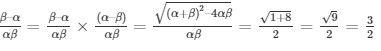7. If one of the zero of the quadratic polynomial f(x) = 4x2 – 8kx – 9 is negative of the other, then find the value of k.

Solution:

From the question, it’s given that:

The quadratic polynomial f(x) where a = 4, b = -8k and c = – 9

And, for roots to be negative of each other, let the roots be α and – α.

So, we can find

Sum of the roots = α – α = -b/a = – (-8k)/1 = 8k = 0 [∵ α – α = 0]

⇒ k = 0

8.  If the sum of the zeroes of the quadratic polynomial f(t)=kt2 + 2t + 3k is equal to their product, then find the value of k.

Solution:

Given,

The quadratic polynomial f(t)=kt2 + 2t + 3k, where a = k, b = 2 and c = 3k.

And,

Sum of the roots = Product of the roots

⇒ (-b/a) = (c/a)

⇒ (-2/k) = (3k/k)

⇒ (-2/k) = 3

∴ k = -2/3

9. If α and β are the zeros of the quadratic polynomial p(x) = 4x2 – 5x – 1, find the value of α2β+αβ2.

Solution:

From the question, it’s given that:

α and β are the roots of the quadratic polynomial p(x) where a = 4, b = -5 and c = -1

So, we can find

Sum of the roots = α+β = -b/a = – (-5)/4 = 5/4

Product of the roots = αβ = c/a = -1/4

To find, α2β+αβ2

⇒ αβ(α +β)

⇒ (-1/4)(5/4) = -5/16

10. If α and β are the zeros of the quadratic polynomial f(t)=t2– 4t + 3, find the value of α4β33β4.

Solution:

From the question, it’s given that:

α and β are the roots of the quadratic polynomial f(t) where a = 1, b = -4 and c = 3

So, we can find

Sum of the roots = α+β = -b/a = – (-4)/1 = 4

Product of the roots = αβ = c/a = 3/1 = 3

To find, α4β33β4

⇒ α3β3 (α +β)

⇒ (αβ)3 (α +β)

⇒ (3)3 (4) = 27 x 4 = 108